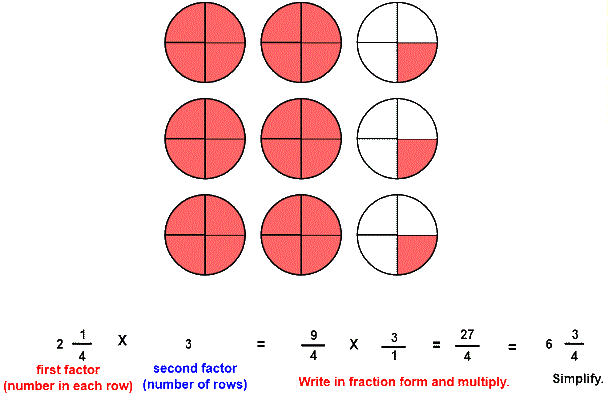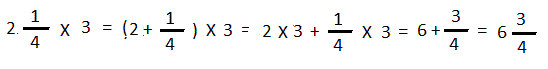# Multiply a mixed number by a whole number

## Multiply a Mixed Number by a Whole Number with Circle Models

MULTIPLY MIXED WITH WHOLE
CORRECT:
ATTEMPTS:
SCORE:
PERCENT
WHOLE
NUMERATOR
DENOMINATOR

# MULTIPLY A MIXED NUMBER BY A WHOLE NUMBER INSTRUCTIONS

Follow the directions in the dialog box after pressing the <START> button. The <EXPLAIN> button may be pressed after you enter the second factor to see how to do the example.

The following picture was made by Multiply with Circles Designer:The parts of a multiplication example are the first factor, the second factor, and the product.

You will notice from the picture that there are 3 rows of circles, each row containing 2 14 circles.

When the program starts, you will be asked to identify the first factor, the number of circles in each row, or 2 14.

You will then be asked to identify the second factor. The second factor is the number of rows, or 3. The second factor in this program will always be 1, 2, or 3.. The program will not continue unless each factor is correctly identified. You will then be asked to identify the product.

You can see from the picture that there are 6 complete circles. The three partial circles can be combined to form 3/4 of a complete circle for a total of 6 34 circles. The product, then, is 6 34.

To calculate the product first write each factor in fraction form as shown in the example above. Then multiply the numerators of each factor for the numerator of the product and the denominators of each factor for the denominator of the product. You may enter the product in fraction form or whole or mixed number form, so 274 or 6 34 are both acceptable.

How to Cancel will play a short animation that shows you this shortcut way to write a fraction in lowest terms.

Since the second factor in each example of MULTIPLY FRACTIONS is a whole number,  you may use another method to calculate the product as shown in the example below. Multiply the whole number part of 2 14 by the second factor 3 and then the fraction part of 3 23 by the second factor 3. Then add the two numbers for the product.

Written out, the example would look like this:As you can see, you are distributing the factor 3 over the whole number and the fraction part of 2 14. When you multiply a factor over two or more addends you are demonstrating the distributive property of multiplication over addition.

For more instruction on multiplying fractions go to How To Multiply Fractions.

After you enter the product you may press the <REPORT> button. The report will ask for your name but you may submit a code for your name. This report will give same results as on the dialog box. The report may be printed or e-mailed.